Next: Blasius Theorem Up: Two-Dimensional Potential Flow Previous: Free Streamline Theory

# Complex Line Integrals

Consider the line integral of some function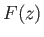of the complex variable taken (counter-clockwise) around a closed curve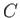in the complex plane: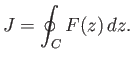(6.158)

Because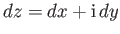, and writing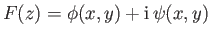, where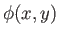andare real functions, it follows that, where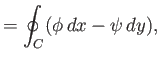(6.159)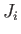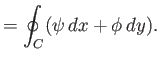(6.160)

However, we can also write the previous expressions in the two-dimensional vector form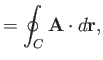(6.161)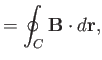(6.162)

where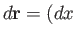,,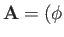,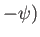, and,. According to the curl theorem (see Section A.22),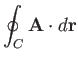(6.163)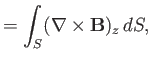(6.164)

whereis the region of the-plane enclosed by. Hence, we obtain(6.165)(6.166)

Let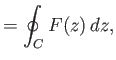(6.167)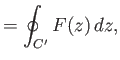(6.168)

where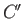is a closed curve in the complex plane that completely surrounds the smaller curve. Consider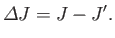(6.169)

Writing, a direct generalization of the previous analysis reveals that(6.170)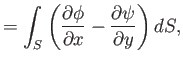(6.171)

whereis now the region of the-plane lying between the curvesand. Suppose thatis well-behaved (i.e., finite, single-valued, and differentiable) throughout. It immediately follows that its real and imaginary components,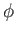and, respectively, satisfy the Cauchy-Riemann relations, (6.17)-(6.18), throughout. However, if this is the case then it is apparent, from the previous two expressions, that. In other words, ifis well-behaved throughoutthen.

The circulation of the flow about some closed curvein the-plane is defined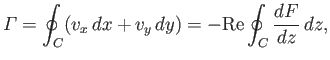(6.172)

whereis the complex velocity potential of the flow, and use has been made of Equation (6.35). Thus, the circulation can be evaluated by performing a line integral in the complex-plane. Moreover, as is clear from the previous discussion, this integral can be performed around any loop that can be continuously deformed into the loopwhile still remaining in the fluid, and not passing over a singularity of the complex velocity,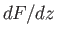.Next: Blasius Theorem Up: Two-Dimensional Potential Flow Previous: Free Streamline Theory
Richard Fitzpatrick 2016-03-31